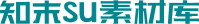SU模型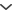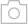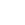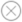• 现代装饰挂画
• 现代风格挂画
• 现代装饰挂画
• 现代装饰挂画
• 现代装饰挂画
• 现代风格挂画 浮雕
• 现代装饰挂画
• 现代装饰挂画
• 现代装饰挂画
• 现代风格装饰挂画 挂画
• 中式字画挂画 书法字画 水墨字画
• 现代装饰挂画 抽象艺术墙饰
• 侘寂装饰挂画
• 侘寂装饰挂画 茶几 花瓶
• 侘寂装饰挂画
• 现代装饰挂画 照片墙
• 新中式装饰画
• 现代装饰挂画
• 侘寂装饰挂画
• 侘寂装饰挂画
• 中式装饰挂画 字画 国画 卷轴 书法字画 水墨字画
• 新中式装饰挂画
• 新中式装饰挂画
• 侘寂装饰挂画
• 现代装饰挂画
• 侘寂装饰挂画 绿植
• 现代装饰挂画
• 侘寂装饰挂画
• 新中式风格挂画 干枝挂画组合
• 欧式挂画组合 装饰画 照片墙 艺术画 抽象画 多联画 墙饰 挂饰 挂件
• 现代装饰挂画
• 现代装饰挂画
• 现代装饰挂画
• 现代风格装饰挂画
• 北欧装饰挂画 照片墙
• 现代装饰挂画
• 侘寂装饰挂画
• 现代装饰挂画
• 现代装饰挂画
• 现代装饰挂画
• 现代装饰挂画
• 新中式挂画
• 现代装饰挂画
• 现代墙饰挂画
• 中式装饰挂画 书法字画 挂画
• 现代装饰挂画
• 美式装饰挂画
• 新中式山水挂画墙饰 装饰画 禅意圆形装饰画 三联幅 四联幅
• 侘寂装饰挂画
• 新中式装饰挂画 立体装饰画 墙面装置艺术
• 现代装饰挂画
• 现代装饰挂画
• 现代装饰挂画
• 新中式装饰挂画
• 侘寂装饰挂画
• 现代装饰挂画
• 现代装饰挂画
• 侘寂装饰挂画
• 现代儿童装饰挂画
• 北欧装饰挂画